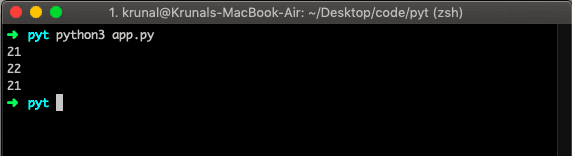# How to Round Numbers in Python

To round numbers in Python, you can use the “round()” function. The round() is a built-in Python function that returns the floating-point number rounded off to the given digits after the decimal point.

### Syntax

``````round(number, digits)
``````

### Arguments

The round() method takes two parameters:

• number – the number that is to be rounded.
• ndigits (Optional) – the number up to which the given number is to be rounded.

### Example 1

``````# for integers
print(round(21))

# for floating point
print(round(21.9))

# even choice
print(round(21.4))
``````

Output### Example 2

To round a number in given n digits, use the “round()” function and pass the ndigits argument.

``````print(round(21.1919, 2))

print(round(21.184646, 3))``````

In the above example, we have passed the second argument, which depends on how many digits you need to round up. In the first example, it is 2; in the second, it is 3. The best use of functions is handling the mismatch between fractions and decimals.

This site uses Akismet to reduce spam. Learn how your comment data is processed.